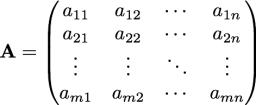# Rectangular 5174

How many columns does a rectangular matrix contain, which contains 45 elements, and the number of its columns is five times larger than the number of its rows?

x =  15

### Step-by-step explanation:Did you find an error or inaccuracy? Feel free to write us. Thank you!

Tips for related online calculators
Are you looking for help with calculating roots of a quadratic equation?
Do you have a linear equation or system of equations and looking for its solution? Or do you have a quadratic equation?